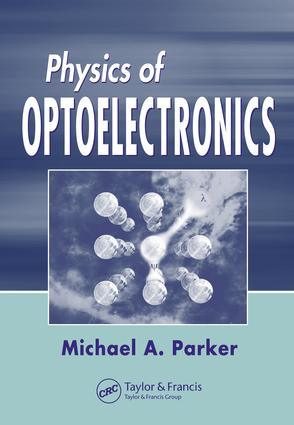# Physics of Optoelectronics

## 1st Edition

CRC Press

798 pages | 371 B/W Illus.

##### Purchasing Options:\$ = USD
Hardback: 9780824753856
pub: 2005-05-23
SAVE ~\$46.00
\$230.00
\$184.00
x
eBook (VitalSource) : 9781315221007
pub: 2018-10-03
from \$28.98

FREE Standard Shipping!

### Description

Physics of Optoelectronics focuses on the properties of optical fields and their interaction with matter. Understanding that lasers, LEDs, and photodetectors clearly exemplify this interaction, the author begins with an introduction to lasers, LEDs, and the rate equations, then describes the emission and detection processes.

The book summarizes and reviews the mathematical background of the quantum theory embodied in the Hilbert space. These concepts highlight the abstract form of the linear algebra for vectors and operators, supplying the "pictures" that make the subject more intuitive. A chapter on dynamics includes a brief review of the formalism for discrete sets of particles and continuous media. It also covers the quantum theory necessary for the study of optical fields, transitions, and semiconductor gain.

This volume supplements the description of lasers and LEDs by examining the fundamental nature of the light that these devices produce. It includes an analysis of quantized electromagnetic fields and illustrates inherent quantum noise in terms of Poisson and sub-Poisson statistics. It explains matter-light interaction in terms of time-dependent perturbation theory and Fermi's golden rule, and concludes with a detailed discussion of semiconductor emitters and detectors.

INTRODUCTION TO SEMICONDUCTOR LASERS

Basic Components and the Role of Feedback

Basic Properties of Lasers

Introduction to Emitter Construction

Introduction to Matter and Bonds

Introduction to Bands and Transitions

Introduction to the pn Junction for the Laser Diode

Introduction to Light and Optics

Introduction to Noise in Optoelectronic Components

Review Exercises

INTRODUCTION TO LASER DYNAMICS

Introduction to the Rate Equations

Stimulated Emission-Absorption and Gain

The Power-Current Curves

Relations for Cavity Lifetime, Reflectance and Internal Loss

Modulation Bandwidth

Introduction to RIN and the Weiner-Khintchine Theorem

Relative Intensity Noise for the Semiconductor Laser

Review Exercises

CLASSICAL ELECTROMAGNETICS AND LASERS

A Brief Review of Maxwell's Equations and the Constituent

Relations Conditions

The Wave Equation

Boundary Conditions for the Electric and Magnetic Fields

Law of Reflection, Snell's Law and the Reflectivity

The Poynting Vector

Electromagnetic Scattering and Transfer Matrix Theory

The Fabry-Perot Laser

Introduction to Waveguides

Physical Optics Approach to Waveguiding

Dispersion in Waveguides

The Displacement Current and Photoconduction

Review Exercises

MATHEMATICAL FOUNDATIONS

Vector and Hilbert Spaces

Dirac Notation and Euclidean Vector Spaces

Hilbert Space

The Grahm-Schmidt Orthonormalization Procedure

Linear Operators and Matrix Representations

An Algebra of Operators and Commutators

Operators and Matrices in Tensor Product Space

Unitary Operators and Similarity Transformations

Hermitian Operators and the Eigenvector Equation

A Relation Between Unitary and Hermitian Operators

Translation Operators

Functions in Rotated Coordinates

Minkowski Space

Review Exercises

FUNDAMENTALS OF DYNAMICS

Introduction to Generalized Coordinates

Introduction to the Lagrangian and the Hamiltonian

Classical Commutation Relations

Classical Field Theory

Schrodinger Equation from a Lagrangian

Linear Algebra and the Quantum Theory

Basic Operators of Quantum Mechanics

The Harmonic Oscillator

Quantum Mechanical Representations

Time Dependent Perturbation Theory

Density Operator

Review Exercises

LIGHT

A Brief Overview of the Quantum Theory of Electromagnetic Fields

The Classical Vector Potential and Gauges

The Plane Wave Expansion of the Vector Potential and the Fields

The Quantum Fields

The Quantum Free-Field Hamilton and EM Fields

Introduction to Fock States

Fockstates as Eigenstates of the EM Hamiltonian

Interpretation of Fock States

Introduction to EM Coherent States

Definition and Statistics of Coherent States

Coherent States as Displaced Vacuum States

Quasi-Orthonormality, Closure and Trace for Coherent States

Field Fluctuations in the Coherent State

Introduction to Squeezed States

The Squeezing Operator and Squeezed States

Some Statistics for Squeezed States

The Wigner Distribution

Measuring the Noise in Squeezed States

Review Exercises

MATTER-LIGHT INTERACTION

Introduction to the Quantum Mechanical Dipole Moment

Introduction to Optical Transitions

Fermi's Golden Rule

Introduction to the Electromagnetic Lagrangian and Field Equations

The Classical Hamiltonian for Fields, Particles and Interactions

The Quantum Hamiltonian for the Matter-Light Interaction

Stimulated and Spontaneous Emission Using Fock States

Introduction to Matter and Light as Systems

Liouville Equation for the Density Operator

The Liouville Equation for the Density Matrix with Relaxation

A Solution to the Liouville Equation for the Density Matrix

Gain, Absorption and Index for Independent Two Level Atoms

Introduction to Jaynes-Cummings' Model

The Interaction Representation for the Jaynes-Cummings' Model

The Master Equation

Quantum Mechanical Fluctuation-Dissipation Theorem

Review Exercises

SEMICONDUCTOR EMITTERS AND DETECTORS

Effective Mass, Density of States and the Fermi Distribution

The Bloch Wave Function

Density of States for Nanostructures

The Reduced Density of States and Quasi Fermi Levels

Fermi's Golden Rule for Semiconductor Devices

Fermi's Golden Rule and Semiconductor Gain

The Liouville Equation and Semiconductor Gain

Review Exercises

APPENDIX 1 REVIEW OF INTEGRATING FACTORS

APPENDIX 2 RATE AND CONTINUITY EQUATIONS

APPENDIX 3 THE GROUP VELOCITY

Simple Illustration of Group Velocity

Group Velocity of the Electron in Free-Space

Group Velocity and the Fourier Integral

The Group Velocity for a Plane Wave

APPENDIX 4 REVIEW OF PROBABILITY THEORY AND

STATISTICS

Probability Density

Processes

Ensembles

Stationary and Ergodic Processes

Correlation

APPENDIX 5 THE DIRAC DELTA FUNCTION

Introduction to the Dirac Delta Function

The Dirac Delta Function as Limit of a Sequence of Functions

The Dirac Delta Function from the Fourier Transform

Other Representations of the Dirac Delta Function

Theorems on the Dirac Delta Functions

The Principal Part

Convergence Factors and the Dirac Delta Function

APPENDIX 6 COORDINATE REPRESENTATIONS OF THE

SCHRODINGER WAVE EQUATION

APPENDIX 7 INTEGRALS WITH TWO TIME SCALES

APPENDIX 8 THE DIPOLE APPROXIMATION

APPENDIX 9 THE DENSITY OPERATOR AND THE

BOLTZMANN DISTRIBUTION

### Subject Categories

##### BISAC Subject Codes/Headings:
SCI055000
SCIENCE / Physics
TEC007000
TECHNOLOGY & ENGINEERING / Electrical
TEC008070
TECHNOLOGY & ENGINEERING / Electronics / Microelectronics
TEC019000
TECHNOLOGY & ENGINEERING / Lasers & Photonics